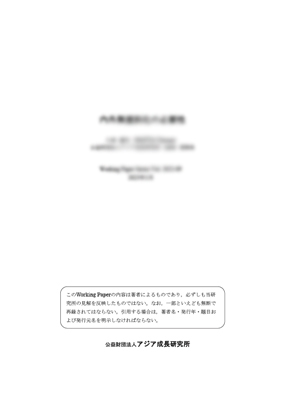# 刊行物

PUBLICATIONS

## Robust goodness-of-fit tests of the classical linear regression model執筆者 Oleksandr Movshuk 2002年 3月 2002-01 1372KB

### 内容紹介

This paper develops two goodness-of-fit tests to verify the joint null hypothesis that regression disturbances have zero mean and constant variance, and are generated from the Gaussian normal distribution. Initially, these tests use a high-breakdown regression estimator to identify a subset of regular observations, and then calculate standardized prediction residuals and studentized prediction residuals, from which the final test statistics are derived. A Monte Carlo study demonstrates that the first test is particularly sensitive to a small number of regression outliers with non-zero mean or unusually large variance, and in general to regression misspecifications that produce regression disturbances with longer tails than could be justified by the normality assumption. In contrast, the second test detects a substantial number of regression outliers, specifications with incorrect functional forms, omissions of relevant variables, and short tails in the distribution of the error term. While most specification tests are designed for a particular alternative, the joint application of the proposed tests has a high power to detect a wide range of breakdowns of the linear regression model. The omnibus property of the suggested tests makes redundant the current practice of running the battery of various specification tests.